Prof. Bryan Caplan

bcaplan@gmu.edu

http://www.bcaplan.com

Econ 812

Week 8: Symmetric Information

I.             Expected Utility Theory

A.           How do people choose between gambles?  In particular, what is the relationship between the value they put on having x with certainty versus having x with p<1?

B.           Simplest theory: Expected value maximization.  People choose whatever option has the highest average monetary value.

1.            Ex: You will be indifferent between (\$1000 with p=.01 and \$1 with p=.99) and \$10.99 with p=1.

C.           This is highly tractable, but also highly unsatisfactory.  Would anyone here really prefer \$1 billion with p=.001 to \$1 million for sure?

D.           This suggests a richer theory of choice under uncertainty, known as expected utility theory (aka von Neumann-Morgenstern expected utility theory).  Intuition: Instead of maximizing average wealth, let us suppose that people maximize expected utility.

E.           Three step procedure:

1.            Assign numerical weights to various outcomes.

2.            Linearly weight outcomes according to their probability.

3.            Choose whatever gamble has the highest linearly weight outcome.

F.            Example.  Suppose I have utility of wealth given by U=W.5.  I can either have a 50% chance of \$10,000 and a 50% chance of \$0, or \$2000 with certainty.  So my expected utility of the first gamble is .5*10,000.5+.5*0.5=50; my expected utility of the second gamble is 1*2000.5=44.72.  Given a choice, then, I would prefer the first option.

G.           Note: Simple utility functions are invariant to any monotonic transformation.  Expected utility functions are not.  (Aside: They are invariant to any affine transformation).

H.           Some implications:

1.            Compounding.  Consumers are indifferent between a 50% chance of a 50% chance of x and a 25% chance of x.

2.            Linearity in probabilities.  If you value a 1% chance of something at \$10, you value a 100% chance at exactly \$1000.

3.            This does NOT however mean that you value \$1000 one hundred times at much as \$10!  It is only the probabilities that matter linearly.

II.            Rational Expectations

A.           As explained in week 1, there are two different interpretations of probability: objective and subjective.

B.           Subjective probability is much more generally applicable than objective probability.

C.           Problem: Subjective probabilities have no necessary connection to reality!  This hardly seems satisfactory.  There is clearly some connection between the real world and what people believe about it.

D.           The leading theoretical effort to formalize the link between subjective probabilities and the real world is known as "rational expectations" or RE.

E.           Simple characterization: A person has RE if judgments are unbiased (mean error is zero) and mistakes are uncorrelated with "available" information.

F.            Deeper characterization: A person has RE if his subjective probability distribution is identical to the objective probability distribution.

G.           Standard modeling technique: everyone is unbiased; information or lack thereof just changes estimates' variance.

H.           RE in no way rules out error; it does not assume that information or cognition is free.

I.             Example #1: Attending graduate school.  No one knows for sure how they will do.  But RE says that on average you correctly estimate how well you will do in the program and what completion will do for you.

J.            Example #2: Renting a movie.  Until you see it, there is no way to know for sure if you will like it.  But RE says that on average your prospective ratings equal your retrospective ratings.  The same goes for your rankings conditional on e.g. movie genre, stars, directors, etc.

K.           Example #3: Wittman on pork barrel spending.

III.          Application: Testing for RE of Economic Beliefs

A.           RE made its first big splash in macro.  In the 1970's, there were many empirical tests performed on e.g. inflation forecasts to check for RE.

B.           How would you go about this?  Try regressing inflation forecasts on a constant and actual inflation:.  RE implies that a=0 and b=1.

C.           One particularly interesting area to me: RE of beliefs about economics.  Most intro econ classes, it seems to me, try to correct students' pre-existing systematic misconceptions.

D.           The Survey of Americans and Economists on the Economy asked economists and the general public identical questions about economics.

E.           Natural test of RE: are the average beliefs of economists and the public identical?  Run the regression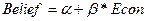, where Econ is a dummy variable =1 for economists and 0 otherwise.  Does b=0?

F.            Of course, this only tests for the public's RE if economists themselves have RE!  Many critics of the economics profession claim that it is the economists who are biased, either because of self-interest or ideology.

G.           These claims are however testable using the SAEE.  Simply re-run the regressioncontrolling for income, job security, ideology, etc, and see if b falls to 0.  (It doesn't).

IV.          Search Theory and Expectational Equilibria

A.           The Arrow-Debreu interpretation of general equilibrium offers one way for economists to analyze economic uncertainty.  But complete contingent claims markets do not seem very realistic.

B.           Is there any other approach?  Yes: there is an extremely general theory of economic action under uncertainty, known as "search theory."

C.           Basic assumptions of search theory:

1.            More time and effort spent "searching" increase your probability of successful discovery.

2.            Searching ability differs between people.

3.            RE. (This can however be relaxed).

D.           Main conclusion: People search so that the marginal cost of searching equals the expected marginal gain of searching.

1.            Qualification: You need to adjust for a searcher's degree of risk-aversion.

E.           The (endless) applications:

1.            Doing R&D.

2.            Hunting and fishing.

3.            Prospecting for gold.

4.            Looking for investment opportunities.

5.            Searching for a job.

6.            Dating.

7.            Rational amnesia.

8.            An economic theory of comedy.

F.            What if people don't search much for a good price?  Then sellers search for consumers.

1.            A tale of Istanbul.

G.           Who is overpaid/underpaid?  Look at who is investing more in search.

H.           Main conclusion:  If the economics of perfect information doesn't make sense, try search theory.  It explains almost everything else.

I.             Some economists, especially Austrians, resist search theory.  Why?  As far as I can tell, it just comes back to objections to probability theory, especially claims that probability theory cannot capture "radical uncertainty" or something along those lines.

V.           Measures of Risk-Aversion

A.           The difference between expected value maximization and expected utility maximization boils down to taste for risk.

B.           Suppose you choose between \$x with p=1 and \$x/q with p=q.  Simplest taxonomy

1.            If you are indifferent between the sure thing and the gamble, you are risk-neutral.

2.             If you prefer the sure thing to the gamble, you are risk-averse.

3.            If you prefer the gamble to the sure thing, you are risk-preferring.

C.           Most economic models assume that actors are risk-averse (though firms are often modeled as risk-neutral).

D.           Graphing: A risk-averse agent has a concave utility of wealth function.  If you draw a line between any two points on the utility function, the utility function is always above that line.  This indicates that a certain payoff of ax+(1-a)y is always preferred to the gamble (x with p=a, y with p=1-a).

E.           Certainty equivalence: If you are indifferent between a gamble and x* with certainty, x* is that gamble's "certainty equivalent."

1.            The risk premium, similarly, is the difference between a gamble's expected value and its certainty equivalent.

F.            There are a number of different ways to quantify risk aversion.  Probably the most common is with the coefficient of absolute risk aversion, which is equal to -u''/u'.  The higher the coefficient, the more risk-averse you are.

G.           Example: If u=w.5, the coefficient of absolute risk aversion is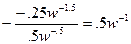In contrast, if u=w, the coefficient is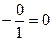, indicating that the latter function is risk neutral.

H.           Note that the coefficient of absolute risk aversion normally decreases with wealth.  This captures the intuition that a millionaire worries less about betting \$1 than someone on the edge of starvation.

VI.          Demand for Insurance

A.           A natural application of the preceding analysis is the demand for insurance.

B.           Specifically, suppose a consumer with EU=w.8 wants to insure his income, which is \$1000 with probability .6 and \$0 with probability .4.  The insurance company offers i worth of insurance - which pays off if the client's uninsured income is \$0 - at price .4xi.  (If x=1, then the price is actuarially fair).

C.           Then the consumer has an EU problem to solve, maximizing wrt i.:D.           Simplify, differentiate and set equal to zero to solve: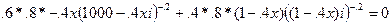E.           This simplifies to: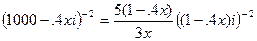F.            Taking the -.2 root of both sides, defining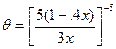.   and solving:G.           Interesting implications: If the insurance contract is actuarially fair,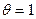, and i*=\$1000; consumers will fully insure.  If the actuarially contract is less than fair, optimal i*<\$1000.

H.           If the price of insurance is high enough, then even risk-averse agents want negative insurance.

VII.        Efficiency Implications of Symmetric Imperfect Information

A.           Many textbooks state that market outcomes are inefficient if there is "imperfect information."  This is a gross over-statement.  Market efficiency and imperfect information are often compatible.

B.           This is particularly clear where there is symmetric imperfect information, where everyone is equally in the dark.

C.           Suppose for example that I don't know how much I will enjoy my consumption bundle, so U(x,y)=xay1-a + e, where e~N(0,s2).  My optimal decision is still to spend a*I on x and (1-a)*I on y.

D.           Similarly, suppose I don't know my relative tastes for x and y, so U(x,y)=xay1-a, where a=.5 with p=.6, and a=.9 with p=.4.  Then I simply maximize U(x,y)=.6[x.5y.5]+.4[x.9y.1].

E.           General point: Just because you are ignorant does not mean you are stupid.  If you are uncertain, you adopt more "general purpose" strategies that take account of all of the possible outcomes.